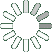Puzzle Command
Daily Puzzle
Number Logic #23
April 5, 2015Find a 6 digit number where:

The 1st digit is the number of 1s in the number. The 2nd digit is an even number. The 3rd digit is an odd number. The 4th digit is an odd number. The 5th digit is the sum of the 3rd and 4th digit. The 6th digit is any digit.

All digits are larger than the previous digit.

Puzzle Notes
6 digit numbers range from 100,000 to 999,999.
Enter in your solution below and click submit.